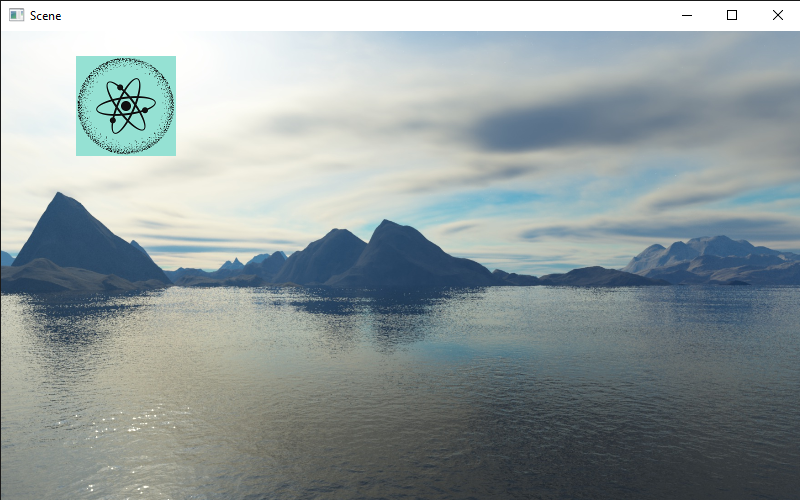# Tutorial 4: 2D¶

This tutorial we will be introducing many new components, namely the `RectTransform` and the `Image2D`. There is more to 2D than this, but most of the tutorial is quite dense in new ideas.

## Data types¶

To facilitate positioning objects, we are going to use `RectAnchors` and `RectOffset`. They both subclass `RectData`, which means they have two properties: `min` and `max`. They are both of type `Vector2`. For now, let’s ignore the `RectAnchors`.

### RectOffset¶

By ignoring `RectAnchors` we can simplify our offset to a literal rectangle. The `min` value specifies the top left corner of the rectangle, and the `max` value specifies the bottom right corner. In PyUnity, the X axis goes left to right and the Y axis goes top to bottom.

For example, a rect that is 100 pixels by 150 pixels, with a top left corner of (50, 75) would be like this:

```>>> offset = RectOffset(
...     Vector2(50, 75),
...     Vector2(150, 225) # 100 + 50 and 150 + 75
... )
```

### RectTransform¶

A `RectTransform` has 5 notable properties: `parent`, `anchors`, `offset`, `rotation` and `pivot`. `parent` is a read-only property, which gets the `RectTransform` of its parent, if it has one. `rotation` is a float measured in degrees, and `pivot` is a point between (0.0, 0.0) and (1.0, 1.0) which defines the rotation point.

### Image2D¶

A `RectTransform` can’t really do much on its own, so we’ll look at the `Image2D` component. This renders a texture in the rect that is defined from the `RectTransform`. If you read tutorial 2, you may have used the `Texture2D` class. Here we can do the exact same:

```>>> gameObject = GameObject("Image")
>>> transform.offset = RectOffset.Rectangle(
...     Vector2(100, 100), center=Vector2(125, 75))
>>> img.texture = Texture2D("python.png")
```

### Canvas¶

All 2D renderers must be a descendant of a `Canvas` element, which can customize the rendering of 2D components. We don’t need to worry about that too much, except that if we were to create an `Image2D` we must make it as a child or descendant of our canvas.

```canvas = GameObject("Canvas")
img = GameObject("Image", canvas)
# And so on...
```

Here the second argument to the `GameObject` constructor specifies its parent, which must be a `GameObject`.

## Code¶

```from pyunity import *

canvas = GameObject("Canvas")

gameObject = GameObject("Image", canvas)
transform.offset = RectOffset.Rectangle(
Vector2(100, 100), center=Vector2(125, 75))
img.texture = Texture2D("pyunity.png")

```

PyUnity image:This is the result:### Interaction¶

The easiest way to create an interactable image is to use the `Button` class. This will trigger whenever any part of the rect is clicked on. Here is an example:

```def callback():
print("Clicked")

# Same canvas and image code as above
...
button.callback = callback
```

If you check the docs for the `Button` class, you can see two more attributes: `state` and `button`. This specifies what state and which button must be pressed for the callback to trigger.

If you would like more control over the button, using a Behaviour is easier as it can interact easily with other GameObjects and is created on a per-component basis. However, if you would like more interaction with the mouse, here is a method:

```class HoverUpdater(Behaviour, GuiComponent):
def HoverUpdate(self):
print("Hovering over component")

# Same canvas and image code as above
...
```

The `GuiComponent` class defines an abstract method called `HoverUpdate` which is called whenever the mouse is hovering over a component. This method will be called exactly once per canvas in a single GuiComponent each frame. In fact, this is how the `Button` class is implemented.

## Anchors¶

For a 2D rect to scale with the window, we can use the `anchors` property of the `RectTransform`. This has two values like the `offset`, a `min` and a `max`. These two values are between `Vector2(0, 0)` and `Vector2(1, 1)`, where 0 and 1 represent the left and right of the window, or the top and bottom of the window. The offsets are applied where the anchors are.

The easiest way to understand this is when the anchors are a single point. For example, the default anchors are `RectAnchors(Vector2(0, 0), Vector2(0, 0))`. This means both points of the anchors are at `Vector2(0, 0)` so all offsets are calculated from the top left.

If we wanted our rect to be centered in the middle at all times, or be offset from the middle, we can set the anchors to be at `Vector2(0.5, 0.5)`. Likewise, if we wanted our rect to be at the bottom right, we can use `Vector2(1, 1)`.

This applies with two anchors: if we wanted our rect to be 50px away from the edges of the window, we would use anchors of `RectAnchors(Vector2(0, 0), Vector2(1, 1))` and offset of `RectOffset(Vector2(50, 50), Vector2(-50, -50))`. This is how we can control the scaling of a rect with respect to the window size.

This tutorial was quite code-heavy, and it is not quite complete. If you are confused, please join our discord support server at https://discord.com/zTn48BEbF9.